# Hausdorffization

Jump to: navigation, search

## Definition

Let$X$ be a topological space. For$a,b \in X$, define$a \sim b$ if any open set containing$a$ intersects any open set containing$b$. The Hausdorffization, also known as Hausdorffification, Hausdorffication, maximal Hausdorff quotient, or Hausdorff quotient, of$X$ is a quotient map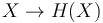$X \to H(X)$ with the universal property that any continuous map from$X$ to a Hausdorff space factors uniquely through the Hausdorffization.

## Iterative construction

### Construction

The Hausdorffization can be obtained through iterative application of the quotient map by an equivalence relation that is described below.

For a topological space$X$. First, consider the relation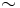$\sim$ on$X$ defined as:$a \sim b$ if for open subsets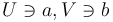$U \ni a, V \ni b$ of$X$,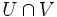$U \cap V$ is non-empty.

Note that the relation above need not itself be an equivalence relation. In particular, it need not be transitive: it is possible that$a \sim b$ and$b \sim c$ but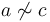$a \not \sim c$.

Denote by$r_X$ the equivalence relation generated by$\sim$. Explicitly,$r_X$ is the subset of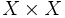$X \times X$ that is the closure in$X \times X$ of the diagonal subspace. Denote by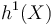$h^1(X)$ the quotient space of$X$ by$r_X$, with$X \to h^1(X)$ the quotient map. In particular, this means that the points of$h^1(X)$ are the equivalence classes in$X$ under$r_X$.

Note that if$X$ is Hausdorff, then$r_X$ is a trivial relation and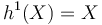$h^1(X) = X$.

We now iteratively define, for every ordinal$\alpha$: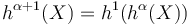$h^{\alpha + 1}(X) = h^1(h^\alpha(X))$

with the composite quotient maps connecting them.

For limit ordinals$\alpha$ we define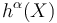$h^\alpha(X)$ as the direct limit of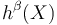$h^\beta(X)$ for$\beta < \alpha$, with the quotient maps between them.

Then,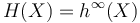$H(X) = h^\infty(X)$.

### Sufficiency of one step for a finite space

If$X$ is a finite space, then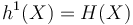$h^1(X) = H(X)$, and moreover, this quotient space is a discrete space.

### Example to illustrate why one step isn't enough

Naively, it might seem that$h^1(X) = H(X)$, because we are already collapsing together any two points that cannot be separated by disjoint open subsets. However, this is not the case. The flaw with this chain of reasoning is that in the new quotient space, we get new open sets that can now start intersecting, even if back in the larger space, the points were separated.

Here is a concrete example of a space$X$:

• The underlying set of$X$ is the union of the set of natural numbers and$\infty$.
• The basis of open subsets is as follows: all sets of the form$\{ 2n - 1 \}$,$[2n - 1, 2n + 1]$, and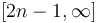$[2n - 1, \infty]$, for$n \in \mathbb{N}$. Qualitatively: the odd numbers all form open subsets, whereas for an even number the smallest open subset containing it comprises that and its two nearest neighbors. And for$\infty$, it does not itself form an open subset but rather its neighborhoods comprise "sufficiently large" numbers. To verify that this is indeed a basis, note that the intersection of any two subsets of the form specified is either again of the form specified or empty.

The relation$\sim$ defined as$a \sim b$ if open subsets containing$a$ and$b$ simplifies to the following:$a \sim b$ if they are both finite and equal, differ by one, or are both even and differ by two.

To see this, note that:

•$\infty$ can be separated from any finite number.
• Two distinct odd numbers can be separated by disjoint open subsets, namely their own singletons.
• Two distinct even numbers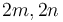$2m, 2n$ with a difference of more than two can be separated by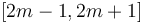$[2m - 1, 2m + 1]$ and$[2n - 1, 2n + 1]$.
• An even number cannot be separated from an adjacent odd number because the smallest open subset containing the even number includes both odd numbers next to it.
• Even numbers that differ by two cannot be separated because the unique smallest open subsets containing the two intersect at the odd number in between.

The equivalence relation generated by$\sim$ has two equivalence classes: all finite numbers form one equivalence class, and$\infty$ forms the other. The quotient space$h^1(X)$ is therefore a two-point space. However, the equivalence class of the point$\infty$ is not an open point in the new space, since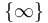$\{ \infty \}$ was not open in$X$. Hence, the new space is not Hausdorff.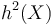$h^2(X)$, which is the one-point space, is indeed Hausdorff and equals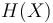$H(X)$.